Description

AekdyCoin loves toys. It is AekdyCoin’s Birthday today and he gets a special “Toy”.

The “Toy” is in bulk and AekdyCoin has to make one by him. Let’s assume that the “Toy” has N small white beads and one Big bead .If someone want to make a “Toy”, he (or she) must always puts the Big bead in center, and then connect the other N small beads around it by using N sticks with equal length, and then the N small beads must be connected by N sticks with equal length, and it could be seen as a regular polygon. Figure 1 shows a “Toy” with 8 small white beads and one big white bead.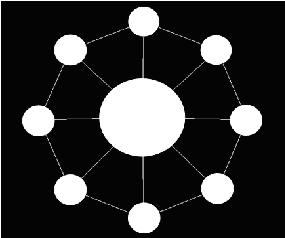Now AekdyCoin has C kinds of available color, say blue, green, yellow, pink …etc. He wants to color these beads, but he thinks that must be too boring and stupid. So he colors these beads with one role: any adjacent beads couldn’t have same color. Figure 2 shows a legal situation, and Figure 3 shows an illegal situation.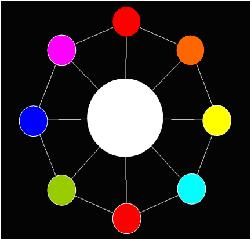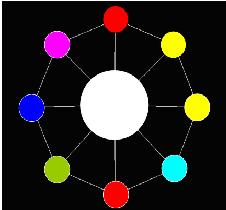It seems that the “Toy” becomes more interesting for AekdyCoin right now; however, he wants to color the big bead in center. Of course, he should follow the role above.

Now AekdyCoin begins to play with the “Toy”, he always colors the big beads and then the other small beads. He should color under the rule above. After several minutes, AekdyCoin finally makes a perfect “Toy”. Figure 4 shows a situation that is under the color rule.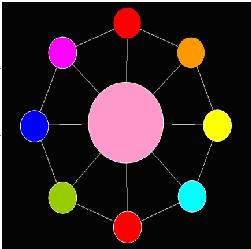AekdyCoin now want to know the different method to color the “Toy” whit at most K color. (“Toy” contains N small beads and one big bead.)

But, no, the problem is not so easy .The repetitions that are produced by rotation around the center of the circular necklace are all neglected. Figure 5 shows 8 “Toy”, they are regard as one method.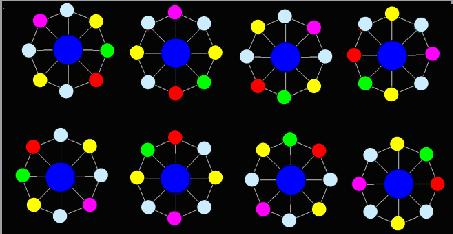Now AekdyCoin will give you N and K, he wants you to help him calculate the number of different methods, because the number of method is so huge, so AekdyCoin just want you to tell him the remainder when divided by M.

In this problem, M = 1,000,000,007.

Input

The input consists of several test cases.(at least 1000)

Every case has only two integers indicating N, K

(3<=N<=10^9, 4<=K<=10^9)

Output

For each case, you should output a single line indicates the remainder of number of different methods after divided by M.

Solutions

POJ2154的加强版。(明明是同一个模型，却转换了那么多次)

对于中间节点的颜色，我们可以固定一种颜色。这样其余的节点都不能选取这种颜色。因此，题目实际上是求本质不同的环染色问题。

对于一个环而言，只有翻转的置换。显然，旋转的置换成群

同时，由POJ2154的结论以及Polya定理可知，最后的答案是$\frac{k}{n} \sum_{i=1}^n f(gcd(i,n))$，转换一下就变成了$\frac{k}{n} \sum_{d \mid n} f(d) \phi(\frac{n}{d})$

乘了一个$k$是因为中间节点有$k$种颜色可以选择；$f(x)$表示$x$个循环，有多少种染色方案可以得到不动点

因此现在的问题就是求出$f(x)$。题目的限制要求是相邻节点不能有相同颜色，因此不能像POJ2154一样直接$k^x$解决。

注意到，若一个置换可以分解成$x$个循环，那么在$mod \, \frac{n}{x}$意义下，一个剩余类可以构成一个循环。这个结论是显然的，因为在每个循环中，只要固定一个最小元素$a$，那么其他元素都可以写成$a+b\frac{n}{x}$的形式。

基于这个结论，若将环按照最小元素排序，那么相邻两个循环不能染同一种颜色。这个是经典的环染色问题，可以直接DP解决。由于$n$很大，因此还需要矩阵乘幂进行优化。

时间复杂度$O(N+\sqrt Nlog \, N)$

同样的，由于约数个数不会太多，因此这个复杂度上界十分宽松。可以看做是$O(\sqrt N log \, N)$